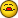Finding criteria within a range

R

Rachel

Hope someone can help me with this query !

I have a spreadsheet with 3 columns of numerical data (with a header row at
the top) which is created by pasting in data from another application. When
the data in one of the columns is within the range 133-137, I want to find
the max and min values within that range. I also want to find the max and
min values in the adjacent columns within the same row range. Obviously I
can do this manually just using max/min functions but I want the data to be
found automatically - so either have a formula in a cell that returns the
result when the data is pasted in, or a macro that can be run to report the
values. The start and end of the range of interest will be different each
time data is pasted in but the total amount of data and the criteria will be
the same.

I am using Excel2002

Thanks in anticipation !

S

ShaneDevenshire

Hi,

If you are willing to use AutoFilters, then instead of using MAX, MIN use
the SUBTOTAL(4,Range) and SUBTOTAL(5,Range). When you apply the auto filter
the max and min of the visible cells is calculated by these formulas. If
necessary you can then copy the results and paste special, value to a
different location.

Alternatively you can use an array formula such as:

=MAX((P120)*(O1:O20>=133)*(O1:O20<=137))

Here the values are in P120, you enter this formula as an array by
pressing Shift Ctrl Enter instead of Enter.

--
Cheers,
Shane Devenshire
Microsoft Excel MVP
help search for life beyond earth.

R

Rachel

Hi Shane

Thanks for your suggestions. The array formula looked better for my needs
so I tried this and was successful at identifying the max value but not the
min.

The formulas I entered (as arrays) were:
=MAX((E2:E721)*(D2721>=133)*(D2721<=137))
This correctly returned the value 2.09
=MIN((E2:E721)*(D2721>=133)*(D2721<=137))
This returned the value 0 which is not correct - the true value is 1.71

Any idea why it's not finding the min?

T

T. Valko

Any idea why it's not finding the min?

Try this array formula** :

=MIN(IF((D2721>=133)*(D2721<=137),E2:E721))

** array formulas need to be entered using the key combination of
CTRL,SHIFT,ENTER (not just ENTER)